# ISEE Upper Level Math : Range

## Example Questions

← Previous 1 3

### Example Question #111 : Data Analysis And Probability

For tonight’s class reading, Erin was assigned to read Chapter 6, which begins on page 60 and ends on page 83. How many pages must Erin read tonight?

23

25

22

24

24

Explanation:

Erin will start reading on page 60 and will stop reading on page 83.

If you subtract these two numbers, you will get the number of pages between 60 and 83: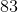-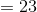However, this subtraction does not include the first page read - page 60. Consider a similar, more simpler case: reading from pages 1 to 3. There are three pages read there: 1, 2, and 3. If you subtract, you get an answer of 2, which leaves you one page short of what you actually read.

So when you subtract 60 from 83, it gives you the pages between those two markers. You need to add on one more page to get your total page count - that is, to include page 60.

Therefore, Erin will read 24 pages.

### Example Question #2 : How To Find Range

Consider the following set of scores from a math test. Give the range of the data.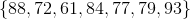Explanation:

The range is a measure of spread. It is the difference between the largest and the smallest data values. So we get: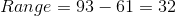### Example Question #3 : How To Find Range

In the following set of data,has the smallest value. If the range of the data values is, give.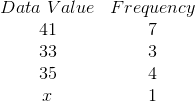Explanation:

The range is a measure of spread. It is the difference between the largest and the smallest data values. So we can write: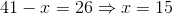### Example Question #4 : How To Find Range

Examine this stem-and-leaf display for a set of data: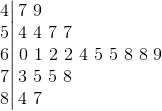What is the midrange of this data set?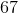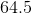Explanation:

The "stem" of this data set represents the tens digits of the data values; the "leaves" represent the units digits.

The midrange of a data set is the arithmetic mean of its greatest and least values, which, in this case, are 47 and 87. Their arithmetic mean is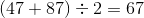, the midrange.

### Example Question #5 : How To Find Range

What is the range of the following set of numbers?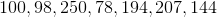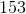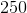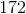Explanation:

To determine the range, first order the numbers in order from least to greatest. Then, find the difference between the greatest number and the least number: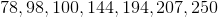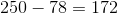Thus, the range is 172.

### Example Question #6 : How To Find Range

What is the range of the following 5 numbers?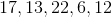Explanation:

The range of a set is determined by subtracting the smallest number from the largest number: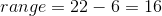### Example Question #7 : How To Find Range

What is the range of the following series of numbers?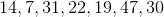Explanation:

Reorder the numbers from smallest to largest:

7, 14, 19, 22, 30, 31, 47

The range is the difference between the largest and smallest values: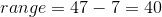### Example Question #8 : How To Find Range

Find the range of the following data set: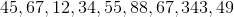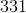Explanation:

Find the range of the following data set:Begin by putting your numbers in increasing order: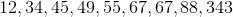Next, find the range by subtracting our first number from our last number: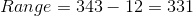### Example Question #9 : How To Find Range

Find the range of the following data set: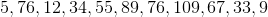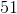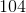Explanation:

Find the range of the following data set:Let's begin by rearranging our terms from least to greatest: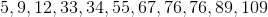Now, find the difference between our largest and smallest terms: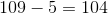### Example Question #10 : How To Find Range

Find the range of the following data set: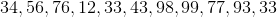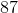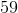Explanation:

Find the range of the following data set:First, let's put our terms in increasing order: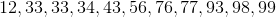Next, our range will just be the difference between the greatest and least terms.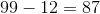So our range is 87.

← Previous 1 3